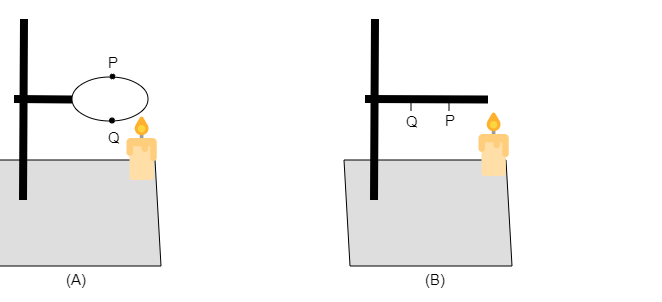# In the arrangements A and B shown in figure, pins P and Q are fixed to a metal loop and an iron rod with the help of wax. In which case are both the pins likely to fall at different times? Explain."

In case $(A)$, the heat will reach $P$ and $Q$ at the same time as the flame is at the same distance from $P$ and $Q$. So, pins $P$ and $Q$ will fall at the same time.

In case $(B)$, the heat will reach $P$ first, as $P$ is nearer than pin $Q$ from the flame. So, the flame will reach pin $P$ in $(B)$. So, pin $P$ will fall first in case $(B)$.